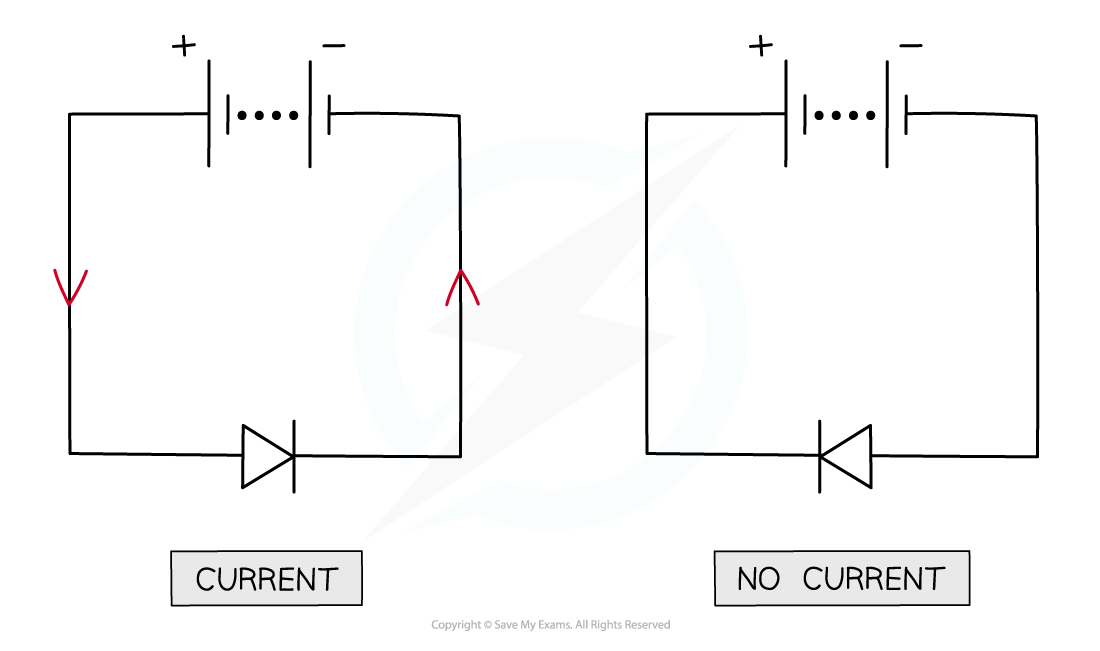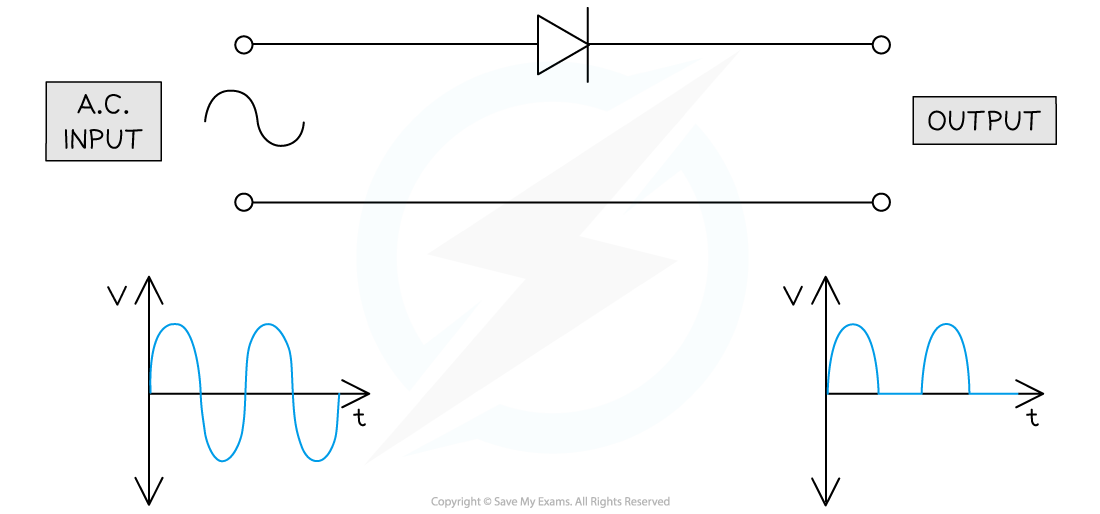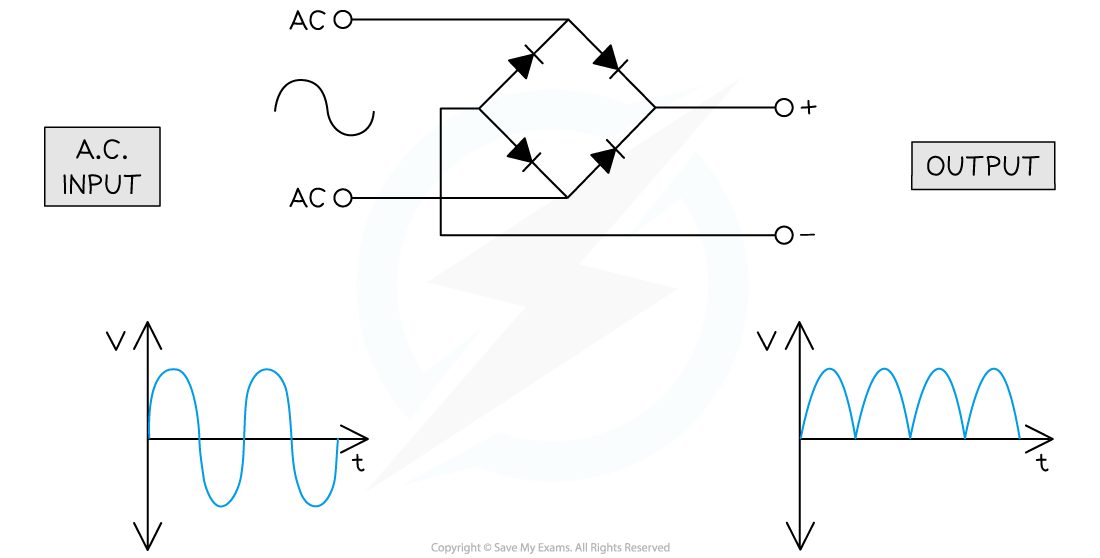# IB DP Physics: HL复习笔记11.2.6 Diode Bridges

### Diode Bridges

• Most electronic devices cannot use alternating current, they require direct current instead
• This is possible by use of a rectifier
• Rectifiers convert alternating current (ac) to direct current (dc)
• There are two types of rectifier:
• The half-wave rectifier
• The full-wave rectifier
• A commonly used rectifier is a diode bridge
• A diode is a component which only allows charge to flow through it in one directionA diode is a component which only allows a current when the potential difference is in the direction of the arrow

• If a power source is connected from the negative to the positive terminal, then there will be no currentIn order to have a current, the diode must point around the circuit from positive to negative

• If a diode is connected to an a.c. (alternating current) power supply, it will only supply a current half of the time leading to the production of direct current
• This is the process of half-wave rectificationA diode can be used to rectify an alternating current into a direct current

• A diode bridge is an arrangement of four (or sometimes more) diodes in a bridge circuit configuration
• When diodes are set up in this way and connected to an a.c. supply, this enables the positive half of each cycle to pass through while the negative half of each cycle is reversed
• This is the process of full-wave rectificationA diode bridge can be used in order to achieve full-wave rectification